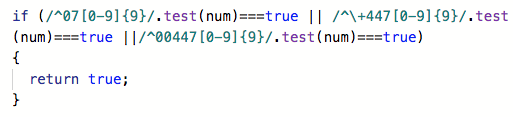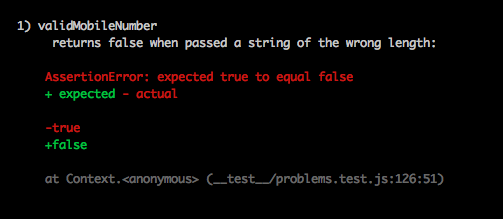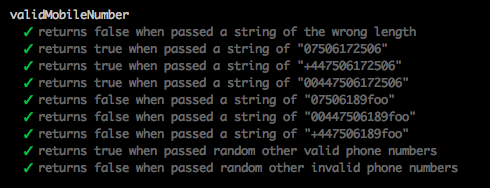# Can't get Regular Expressions to work

any idea why ?

``````
function replaceLettersWithDashes (x)
{

var array1 = x.split('')
var array2 = []

for(i=0;i<array1.length;i++)
{
if(x[i]===/\p/)
{
array2.push('');
}

else if(x[i]=== /\W/)
{
array2.push(x[i])
}

else if (x[i]=== /\w/)
{
array2.push('-')
}

else
{
array2.push('-')
}

}

console.log(array2)

}

replaceLettersWithDashes ('@ ~')

`````````

What is the expected output of the call replaceLettersWithDashes (’@ ~’)

I want it to replace alphabetic letter with dashes, everything else should return as is

If all you want to do is replace letters with dashes, then I would simply use:

``````function replaceLettersWithDashes(x) {
return x.replace(/[a-z]/gi, '-');
}
``````
1 Like

Im just concerned as to why the regular expressions dont seem to be working?

Because you are not using the correct syntax. For example, you wrote the following:

``````else if (x[i]=== /\w/)
``````

You are asking if a specific element value in the x array is equal to a regular expression object. You are not asking if a specific element value in the x array is a word character, which is what I think you are trying to do with this else if statement.

If you really want to use a regular expression in this particular else if statement, then take a look at https://developer.mozilla.org/en-US/docs/Web/JavaScript/Reference/Global_Objects/RegExp/test for a way to do that.

If you can not figure it out, then take a peak at the following code:

``````else if (/\w/.test(x[i])
``````

Just keep in mind that a word character is defined as a character from a-z, A-Z, 0-9, including the _ (underscore) character.

Unsure whats going on here, the num ‘073’ is being read as ‘59’ . Not sure if this is the only thing wrong.

``````function validMobileNumber (num) {

console.log(num);
console.log(typeof num)

if (/^07[0-9]{9}/.test(num)===true)
{
return true;
}

if(/^\+447[0-9]{9}/.test(num)===true )
{
return true;
}

if(/^00447[0-9]{9}/.test(num)===true)
{
return true
}

return false;
}

``````

When you add a leading zero to a decimal integer number, the JavaScript interpreter thinks it is an octal (a number in base 8) instead of a decimal (a number in base 10). 073 in base 8 is 59 in decimal.

Im testing the string ‘0750617250638’ againstElse it returns false, not sure whats upIt is always better if you can post actual code rather than a screenshot. It allows those trying to help you work with the actual code and not have to retype it.

``````function validMobileNumber (num) {

num = String(num);
console.log(num);
console.log(typeof num)

if (/^07[0-9]{9}/.test(num)===true || /^\+447[0-9]{9}/.test(num)===true ||/^00447[0-9]{9}/.test(num)===true)
{
return true;
}

if(/^\+447[0-9]{9}/.test(num)===true )
{
return true;
}

if(/^00447[0-9]{9}/.test(num)===true)
{
return true
}

return false;
}

validMobileNumber('0750617250638')

``````

This is what I am testing this against

``````escribe('validMobileNumber', function () {
it('returns false when passed a string of the wrong length', function () {
expect(validMobileNumber('123')).to.equal(false);
expect(validMobileNumber('0750617250638')).to.equal(false);
expect(validMobileNumber('+447712368768724988')).to.equal(false);
});
it('returns true when passed a string of "07506172506"', function () {
expect(validMobileNumber('07506172506')).to.equal(true);
});
it('returns true when passed a string of "+447506172506"', function () {
expect(validMobileNumber('+447506172506')).to.equal(true);
});
it('returns true when passed a string of "00447506172506"', function () {
expect(validMobileNumber('00447506172506')).to.equal(true);
});
it('returns false when passed a string of "07506189foo"', function () {
expect(validMobileNumber('07506189foo')).to.equal(false);
});
it('returns false when passed a string of "00447506189foo"', function () {
expect(validMobileNumber('00447506189foo')).to.equal(false);
});
it('returns false when passed a string of "+447506189foo"', function () {
expect(validMobileNumber('+447506189foo')).to.equal(false);
});
it('returns true when passed random other valid phone numbers', function () {
expect(validMobileNumber('00447555123456')).to.equal(true);
expect(validMobileNumber('+447676111222')).to.equal(true);
expect(validMobileNumber('07898888643')).to.equal(true);
expect(validMobileNumber('07766555432')).to.equal(true);
expect(validMobileNumber('07989765490')).to.equal(true);
});
it('returns false when passed random other invalid phone numbers', function () {
expect(validMobileNumber('004475551&&&23456')).to.equal(false);
expect(validMobileNumber('-447676111222')).to.equal(false);
expect(validMobileNumber('09898888643')).to.equal(false);
expect(validMobileNumber('+449166555432')).to.equal(false);
expect(validMobileNumber('00448989765493')).to.equal(false);
expect(validMobileNumber('cats')).to.equal(false);
});
});
``````

The first condition of the first if statement evaluates to true, so it is going to return true. I assume you already know that? Do you understand why it evaluates to true?

It evaluates to true, because the first regular expression asks the following:

2. Are there 9 digits which follow the ‘07’? Yes, the digits are ‘506172506’

1 Like

aaaaah so its testing for 9 or more rather than exactly 9 ok

No, it is testing for exactly 9 following the ‘07’ and it finds exactly 9 digits (506172506). Think about another special character like the ^ you are using in front of the 07 which would mark the end of the string.

oh so like \b to end it Ill check it out

``````^07[0-9]{9}\b
``````

That is not the one I was thinking about, but it should work also.

Thanks!FYI - Your function could have been written as:

``````function validMobileNumber (num) {
num = String(num);
return /^(07|(\+|00)447)\d{9}\$/.test(num);
}
``````

If num will always be passed in as a string, you could remove the num = String(num); line.

1 Like